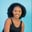Related Tags

python3
pandas

# How to use the Series.agg() function in pandasMaria Elijah

## The Series.agg() function

The pandas Series.agg() function applies a function or list of functions to the elements in a series along a given axis and returns the result.

### Syntax

Series.agg(func, axis=0)


### Parameters:

• func: It represents the function or list of functions used to perform operations on the series elements.
• axis: It indicates the axis in which the operation is to be performed. The value 0 and 1 indicate rows and columns, respectively. The default is 0.

Note: If a list of functions is used, the Series.agg() function returns multiple results.

## Examples

The following code will demonstrate how to use the Series.agg() function in pandas.

### Example 1: Use a single function

import pandas as pd

# create Series
my_series = pd.Series([17, 4, 28, 15, 23, 7, 9])

# multiple each element in the by the power of 2
# using agg() and passing the lambda function
print(my_series.agg(lambda x: x **2))

### Explanation

In the code above:

• Line 1: We import the pandas library.

• Lines 4: We create the Series, my_series.

• Line 8: We use the agg() function, then we pass a lambda function to multiple each element in the Series by the power of 2.

### Example 2: Use a list of functions

import pandas as pd

# create Series
my_series = pd.Series([17, 4, 28, 15, 23, 7, 9])

# sum the element in the series
# using agg() and passing the sum method
print(my_series.agg([min, sum, len, max]))

### Explanation

In the code above:

• Line 1: We import the pandas library.

• Lines 4: We create a series my_series.

• Line 8: We use the agg() function, then we pass a list of functions:

• The min function finds the minimum element in the Series.
• The sum function finds the minimum element in the Series.
• The len function finds the len of the Series.
• The max function finds the maximum element in the Series.

Note: Each function is enclosed in a square bracket and separated by a comma. Also, you can add as many functions as needed.

RELATED TAGS

python3
pandas

CONTRIBUTORMaria Elijah
RELATED COURSES

View all Courses

Keep Exploring

Learn in-demand tech skills in half the time